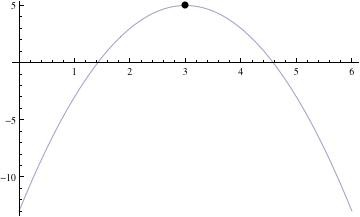Find the vertex of the parabola. Y = -2x^2 + 12x - 13?

For the parabola
y = ax^2 + bx + c
The x value of the vertex is given by
x = -b/(2a)
For your parabola, this is
x = -(12)/(2*(-2)) = 3
The value of y at that point is
y = -2(3^2) + 12(3) - 13
= -2*9 + 36 - 13
= 5
The vertex is located at (x, y) = (3, 5).thanked the writer.